Published: 15 August 2017

# Research on vibration localization of mistuned bladed disk system

Hongyuan Zhang1
Huiqun Yuan2
Wenjun Yang3
Tianyu Zhao4
1School of Automobile and Traffic, Shenyang Ligong University, Shenyang, China
1, 3, 4School of Mechanical Engineering and Automation, Northeastern University, Shenyang, China
2College of Sciences, Northeastern University, Shenyang, China
Corresponding Author:
Huiqun Yuan
Views 187

#### Abstract

In order to assess the vibration localization influences of different parameters of structural of the mistuned bladed disk system, the pre-stressed component mode synthesis method was adopted, and reduced-order models (ROMs) of the aero-engine compressor bladed disk system were built. Identification of the mistuning parameter was set based on the combination of blade static frequency, dichotomy and finite element analysis method. By Defining the vibration localization factor, the influence parameters such as the engine orders of excitation, mean frequency and stiffness ratio were considered, localization characteristics of the vibration response was analyzed, and the influence rule of different parameters on the vibration response were discussed. The result shows that the engine orders of excitation has significant influences on the frequency, displacement and strain energy distribution, with the increase of the engine orders of excitation, the relative localization factors firstly increase, and then decrease; the relative localization characteristics of the bladed disk system on the different mean frequencies were found out; with the increase of the stiffness ratio of the blade and disk, maximum vibration amplitude and strain energy of the bladed disk system gradually decline.

## 1. Introduction

The aero-engine compressor bladed disk system should be a circumferential cyclic symmetry structure in theory, however, due to the variance of manufacture, assembling, wear and chatter suppression, the difference on quality, stiffness and natural frequency between the blades were caused, that was called mistuning. Mistuning will cause the vibration energy of the bladed disk system to concentrate on few blades, it causes the serious localization phenomenon, moreover, it results in fatigue failure, and then impacts the service life of the compressor bladed disk system, so a research on vibration localization of the mistuned bladed disk system becomes particularly important.

The pre-stressed component mode synthesis method is adopted the finite element reduced-order models (ROMs) for the compressor bladed disk system are built. Mistuning parameter identification is based on the blade static frequency, dichotomy and finite element analysis. The influence parameters such as the engine orders of excitation, mean frequency and stiffness ratio are considered, the relative vibration localization factors are defined, as well as the influences of different parameters are discussed.

## 2. Pre-stressed component mode synthesis method

 Blade material property Property value Disk material property Property value Mass density 4400 kg·m−3 Mass density 4700 kg·m−3 Modulus of elasticity 113 GPa Modulus of elasticity 150 GPa Poisson’s ratio 0.3 Poisson’s ratio 0.3

Fig. 1Finite element model of bladed disk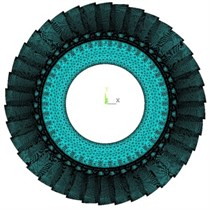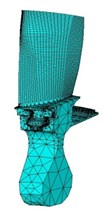The pre-stressed component mode synthesis method enables the inner coordinates of each substructure to be as the slave degree of freedom and the interfacial coordinate of each substructure to be as the master degree of freedom. The dynamic reduced-order technology is adopted for condensing the slave degree of freedom of each substructure on the interfacial master degree of freedom, interfacial displacement compatibility conditions and interface force equilibrium condition are utilized to integrate the reduced super-element to form the whole system. The general non-damping free vibration equation of the $i$th substructure finite element model can be written as:

1
$\begin{array}{ll}{M}_{i}{\stackrel{¨}{q}}_{i}+{K}_{i}{q}_{i}=0,& \left(i=1,2,\dots ,38\right)\end{array},$

where ${M}_{i}$ and ${K}_{i}$ is the mass matrix and the stiffness matrix, accordingly, and ${q}_{i}$ shows the displacement vector. The stress state of the aero-engine compressor blade thin in one or two degrees of freedom will influence the natural frequency and the dynamic response of the structure under the action of centrifugal force, in consideration of the influences of centrifugal rigidization on the dynamic characteristics of the rotor system, linear stress analysis is conducted under the condition of the static state to translate rotating centrifugal load into pre-stressed force effect matrix $S$ of the structure to be annexed in the kinetic equation, therefore, the obtained kinetic equation by considering the centrifugal stiffening effect is:

2
$\begin{array}{ll}{M}_{i}{\stackrel{¨}{q}}_{i}+{K}_{i}^{\text{'}}{q}_{i}=0,& \left(i=1,2,\dots ,38\right)\end{array},$

where ${K}_{i}^{\text{'}}$ is the stiffness matrix after considering the pre-stressed force effect matrix. The displacement vector and coefficient matrix in the Eq. (2) are divided into the master degree of freedom ${q}_{m}$on the boundary and the slave degree of freedom ${q}_{s}$ on the non-boundary (subscript $m$ and subscript $s$ respectively show the master degree of freedom and the slave degree of freedom):

3
$\left[\begin{array}{ll}{M}_{mm}& {M}_{ms}\\ {M}_{sm}& {M}_{ss}\end{array}\right]\left[\begin{array}{l}{\stackrel{¨}{q}}_{m}\\ {\stackrel{¨}{q}}_{s}\end{array}\right]+\left[\begin{array}{ll}{K}_{mm}^{\text{'}}& {K}_{ms}^{\text{'}}\\ {K}_{sm}^{\text{'}}& {K}_{ss}^{\text{'}}\end{array}\right]\left[\begin{array}{l}{q}_{m}\\ {q}_{s}\end{array}\right]=\left[\begin{array}{l}{f}_{i}\\ 0\end{array}\right],$

where ${f}_{i}$ is the interface force. When the interface of substructure completely fixed, that is ${q}_{m}=0$, the equation of motion of the fixed boundary condition can be written as:

4
$\left[{M}_{ss}\right]\left\{{\stackrel{¨}{q}}_{s}\right\}+\left[{K}_{ss}^{\text{'}}\right]\left\{{q}_{s}\right\}=0.$

The regularization mode set $\left[{\varphi }_{1}\right]$ can be solved from equation, it consists of the low order mode set $\left[{\varphi }_{l}\right]$ and the high order mode set $\left[{\varphi }_{h}\right]$ as follow:

$\left[{\varphi }_{1}\right]=\left[{\varphi }_{l}\right]\left[{\varphi }_{h}\right]$, the mode set yields:

5
${\left[{\varphi }_{1}\right]}^{T}\left[{M}_{ss}\right]\left[{\varphi }_{1}\right]=E,$
6
${\left[{\varphi }_{1}\right]}^{T}\left[{K}_{ss}^{\text{'}}\right]\left[{\varphi }_{1}\right]=\left[\mathrm{\Lambda }\right]=\left[\begin{array}{ll}{\mathrm{\Lambda }}_{l}& \\ & {\mathrm{\Lambda }}_{h}\end{array}\right],$

where $\left[{\mathrm{\Lambda }}_{l}\right]=\mathrm{d}\mathrm{i}\mathrm{a}\mathrm{g}\left({\lambda }_{1}^{2},{\lambda }_{2}^{2},\cdots ,{\lambda }_{l}^{2}\right)$, $\left[{\mathrm{\Lambda }}_{h}\right]=\mathrm{d}\mathrm{i}\mathrm{a}\mathrm{g}\left({\lambda }_{l+1}^{2},{\lambda }_{l+2}^{2},\cdots ,{\lambda }_{h}^{2}\right)$, $\lambda$ is characteristic values, $E$ is identity matrix.

Let ${\varphi }_{m}$ be ${\varphi }_{1}$, that is:

7
$\left[{\varphi }_{m}\right]=\left[{\varphi }_{1}\right],$

where $\left[{\varphi }_{m}\right]$ is master modes set of substructure.

It is assumed that the inertia term is not considered, and the force is only on the master degree of freedom, the Eq. (8) can be obtained by Eq. (3):

8
$\left[\begin{array}{ll}{K}_{mm}^{\text{'}}& {K}_{ms}^{\text{'}}\\ {K}_{sm}^{\text{'}}& {K}_{ss}^{\text{'}}\end{array}\right]\left\{\begin{array}{l}{q}_{m}\\ {q}_{s}\end{array}\right\}=\left\{\begin{array}{l}{f}_{i}\\ 0\end{array}\right\}.$

So that the Eq. (8) can be written as:

$\left[{K}_{sm}^{\text{'}}\right]\left\{{q}_{m}\right\}+\left[{K}_{ss}^{\text{'}}\right]\left\{{q}_{s}\right\}=0$

Thus, the ${q}_{s}$ can be written as:

$\left\{{q}_{s}\right\}=-{\left[{K}_{ss}^{\text{'}}\right]}^{-1}\left[{K}_{sm}^{\text{'}}\right]\left\{{q}_{m}\right\}.$

Let ${q}_{m}$ be $E$ and ${q}_{s}$ be ${\varphi }_{J}$, that is:

$\left\{{q}_{m}\right\}=\left[E\right],\left\{{q}_{s}\right\}=\left[{\varphi }_{J}\right].$

Then:

$\left[{\varphi }_{J}\right]=-{\left[{K}_{ss}^{\text{'}}\right]}^{-1}\left[{K}_{sm}^{\text{'}}\right]\left[E\right]=-{\left[{K}_{ss}^{\text{'}}\right]}^{-1}\left[{K}_{sm}^{\text{'}}\right].$

Now we define $\left[{\varphi }_{c}\right]$ the mode set of constraints, can be written as:

9
$\left[{\varphi }_{c}\right]=\left[\begin{array}{l}E\\ {\varphi }_{J}\end{array}\right]=\left[\begin{array}{c}E\\ -{{K}_{ss}^{\text{'}}}^{-1}{K}_{sm}^{\text{'}}\end{array}\right].$

Ritz vectors can consist of $\left[{\varphi }_{m}\right]$ and $\left[{\varphi }_{c}\right]$, that is:

10
$\left[{\varphi }_{i}\right]={\left[{\varphi }_{c}{\varphi }_{m}\right]}_{i}={\left[\begin{array}{ll}E& 0\\ {\varphi }_{J}& {\varphi }_{1}\end{array}\right]}_{i}={\left[\begin{array}{cc}E& 0\\ -{K}_{ss}^{\text{'}-1}{K}_{sm}^{\text{'}}& {\varphi }_{1}\end{array}\right]}_{i}.$

Make use of coordinate transformation equation:

11
${q}_{i}={\varphi }_{i}{\left[\begin{array}{c}{p}_{m}\\ {p}_{s}\end{array}\right]}_{i},$

where $p$ is the substructure modal coordinates; ${\varphi }_{i}$ is the coordinate transformation matrix  of the fixed interface method, ${\varphi }_{1}$ is the eigenvector of the substructure boundary node under the fixed condition, $E$ is the unit matrix, and 0 is null matrix. The kinetic equation expressed as the Eq. (2) by utilizing the Eq. (11) is transformed to the modal coordinate:

12
$\begin{array}{ll}{\stackrel{-}{M}}_{i}{\stackrel{¨}{q}}_{i}+{\stackrel{-}{K}}_{i}{q}_{i}=0,& \left(i=1,2,\dots ,38\right),\end{array}$

where ${\stackrel{-}{M}}_{i}={\varphi }_{i}^{T}{M}_{i}{\varphi }_{i}$, ${\stackrel{-}{K}}_{i}={\varphi }_{i}^{T}{K}_{i}^{\text{'}}{\varphi }_{i}$.

The degree of freedom reduction method  is utilized, let ${\varphi }_{m}$ be ${\varphi }_{1}^{\text{'}}$, that is:

13
$\left[{\varphi }_{m}\right]=\left[{\varphi }_{1}^{\text{'}}\right]=\left[{\varphi }_{l}\right],$
14
${\varphi \text{'}}_{i}={\left[{\varphi }_{c}{\varphi }_{m}\right]}_{i}={\left[\begin{array}{ll}E& 0\\ {\varphi }_{J}& {\varphi }_{1}^{\text{'}}\end{array}\right]}_{i}={\left[\begin{array}{cc}E& 0\\ -{K\text{'}}_{ss}^{-1}{K\text{'}}_{sm}& {\varphi }_{1}^{\text{'}}\end{array}\right]}_{i}{\left[\begin{array}{cc}E& 0\\ -{K\text{'}}_{ss}^{-1}{K\text{'}}_{sm}& {\varphi }_{l}\end{array}\right]}_{i},$

where ${\varphi }_{1}^{\text{'}}$ is the low order modal set truncated by high order, so:

15
${q}_{i}={\varphi }_{i}^{\text{'}}{\left[\begin{array}{c}{p}_{m}\\ {p}_{a}\end{array}\right]}_{i},$

where ${p}_{a}$ is the reduced-order modal coordinate of the slave degree of freedom ${p}_{s}$; so, the modal coordinate vector in the Eq. (12) changes into ${p}_{i}={\left[\begin{array}{ll}{p}_{m}& {p}_{a}\end{array}\right]}_{i}^{T}$.

In consideration of rigid connection among the substructures, the non-independent coordinate $P={\left[\begin{array}{llll}{p}_{1}^{T}& {p}_{2}^{T}& \cdots & {p}_{38}^{T}\end{array}\right]}_{i}^{T}$ is converted into the generalized coordinate of the overall bladed disk system by using the following transformation of coordinates :

16
$P=\beta X.$

Thus, to establish the free vibration equation as:

17
$\begin{array}{ll}M\stackrel{¨}{X}+KX=0,& \left(i=1,2,\dots ,38\right)\end{array},$

where $M$ and $K$ are less than the mass matrix and stiffness matrix under the original system generalized coordinate because of the reduction of degree of freedom.

In the Eq. (17), because of the neglected high order mode, so $M$ and $K$ are less than the mass matrix and stiffness matrix under the original system generalized coordinate through the reduction of degree of freedom. The choice of mode truncation number is especially important for the accuracy of the analysis. The vibration characteristics of the bladed disk at different mode truncated numbers are calculated by pre-stressed component mode synthesis method.

As shown in Fig. 2, $T$ represents the mode truncation number, when $T$ is equal to 5 and 10, the natural frequency is calculated to be far from the global method, with the increase of the mode truncation number, the accuracy is further improved, when $T$ is equal to 15 and 20, the accuracy is higher. When $T$ is equal to 40 and 60, the calculated values of natural frequencies are in good agreement with the global method; It shows that the PCMS method is convergent.

Fig. 2Comparison of Global method with pre-stressed component mode synthesis method by different mode truncation numbers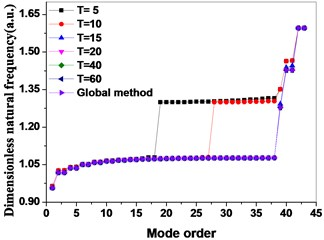## 3. Identification of mistuned parameter

For the engineering actual bladed disk system, mistuning destroys the cyclic symmetry and causes the stiffness and frequency of the blades to be different, and for the test frequency distribution, it is important to correctly identify the mistuned parameters for analyzing the mistuned bladed disk system. The stiffness and the frequency of the mistuned blades are simulated by introducing different disturbance factor ${P}_{j}$ to the elastic modulus of the blades, that is:

18
$\begin{array}{ll}{E}_{j}={E}_{0}\left(1+{P}_{j}\right),& j=1,2,\dots ,38.\end{array}$

In the Eq. (18), ${E}_{j}$ is the elastic modulus of each blade, ${E}_{0}$ is the elastic modulus of the tuned blade, and ${P}_{j}$ is the perturbation parameters.

For the identification of mistuned parameters, the method based on the combination of the blade static frequency, dichotomy and finite element analysis are adopted: firstly, a static frequency test is conducted on the blades of the compressor bladed disk system to obtain the first-order bending static frequency of each blade; secondly, the method of combining dichotomy and finite element analysis are applied to identify the mistuned elastic modulus corresponding to the natural frequency for first-order bending of the blade static frequency test by introducing different perturbation parameters ${P}_{j}$. Two kinds of mistuned aligning are provided for two groups of blade frequency test data, as shown in Table 2. The dimensionless data is the ratio of mistuned blade data to tuned blade data. The basic flow of the blade mistuned parameter identification based on the combination of blade static frequency, dichotomy and finite element analysis is shown in Fig. 3. Blade meshes choose SOLID45 element type and tenon mesh choose SOLID187 element type, blade and tenon are divided into 35072 elements, and the number of nodes is 49051. The blade finite element model is shown in Fig. 4, and the full constraint is implemented on a contact surface between the tenon and mortise. The specific identification steps are as follows: firstly, the natural frequency for first-order bending of the blade corresponding to the top and bottom limitation of the elastic modulus is calculated; secondly, through dichotomy iterative computations, the statics frequency for first-order bending measured from the blade statics frequency test is compared with the finite element analysis result of the natural frequency for first-order bending of the blade, the calculation convergence standard is that the error is less than 0.01, and then the mistuned elastic modulus of single blade corresponding to the statics frequency of the blade test is provided. The elastic modulus mistuning corresponding to the natural frequency for first-order bending of the blade statics frequency obtained through mistuning identification is shown in Fig. 5.

Table 2Dimensionless test frequency of mistuned blades (a.u.)

 Blade number Dimensionless test frequency of group 1 Dimensionless test frequency of group 2 Blade number Dimensionless test frequency of group 1 Dimensionless test frequency of group 2 Pattern 1 Pattern 2 Pattern 3 Pattern 4 Pattern 1 Pattern 2 Pattern 3 Pattern 4 1 0.9695 0.9695 0.9695 0.9695 20 1.0076 1.0076 1.0061 1.0061 2 1.0229 1.0229 1.0229 1.0244 21 0.9954 0.9954 0.9832 0.9832 3 0.9679 0.9679 0.9847 0.9847 22 1.0092 1.0092 1.0061 1.0061 4 0.9969 0.9969 1.0076 1.0076 23 0.9954 0.9954 0.9832 1.0229 5 0.9847 0.9847 0.9847 0.9847 24 1.0076 1.0076 1.0061 1.0061 6 0.9985 0.9985 1.0076 1.0076 25 0.9939 0.9939 0.9832 0.9832 7 0.9863 0.9863 0.9847 1.0229 26 1.0305 1.0305 1.0061 1.0061 8 0.9985 1.0382 1.0076 1.0076 27 0.9786 0.9786 0.9695 0.9695 9 0.9847 0.9847 0.9847 0.9847 28 1.0290 1.0290 1.0229 1.0244 10 0.9969 0.9969 1.0076 1.0076 29 1.0015 1.0015 0.9832 0.9832 11 0.9847 0.9847 0.9847 0.9847 30 1.0137 1.0137 1.0061 1.0061 12 1.0000 1.0000 1.0076 1.0076 31 0.9969 0.9969 0.9832 0.9832 13 0.9863 0.9863 0.9847 0.9847 32 1.0107 1.0260 1.0061 1.0061 14 1.0244 1.0244 1.0076 1.0076 33 0.9954 0.9725 0.9863 1.0229 15 0.9710 0.9710 0.9695 0.9695 34 1.0092 1.0092 1.0092 1.0092 16 1.0244 1.0244 1.0229 1.0244 35 0.9954 0.9954 0.9863 0.9863 17 0.9969 0.9969 0.9847 0.9847 36 1.0076 1.0076 1.0092 1.0092 18 1.0092 1.0092 1.0061 1.0061 37 0.9954 0.9954 0.9863 0.9863 19 0.9954 0.9954 0.9847 0.9847 38 1.0076 1.0076 1.0092 1.0092

Fig. 3Flow chart of mistuned parameter identification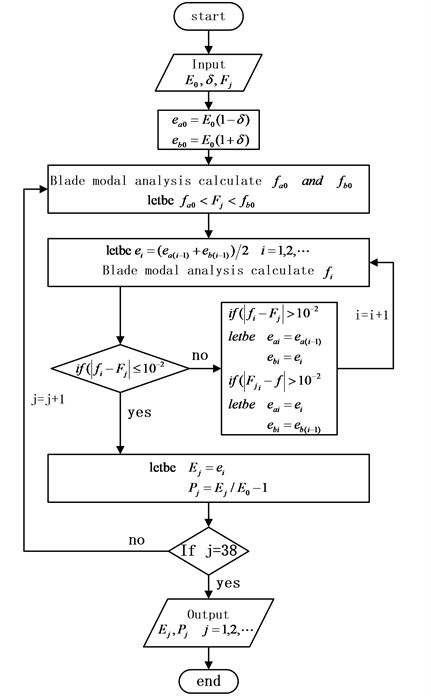Fig. 4Finite element model of bladed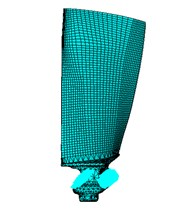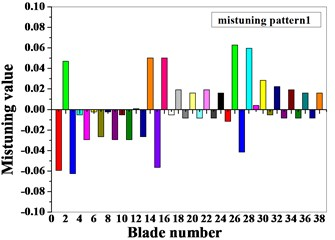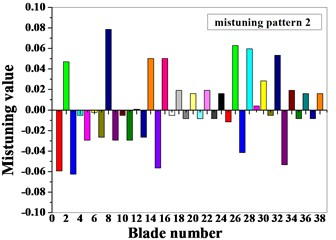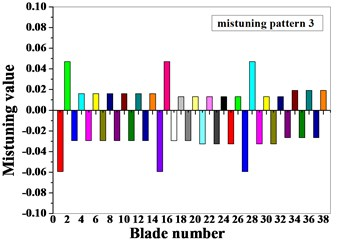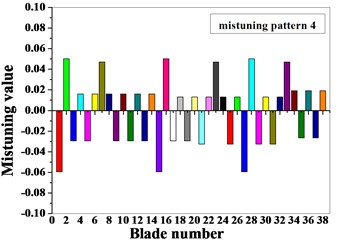## 4. Localization influences of different influences on mistuned bladed disk

The natural frequencies of the bladed disk system are equal to the exciting force frequency, and the resonance occurs when the engine orders of excitation is equal to the nodal diameter. The bladed disk mistunes to cause the vibration mode not to conform to the development form of the harmonic and to expand to the different nodal diameter with mistuning, and the level of sensitivity of the vibration mode on different orders to mistuning is different. Therefore, it is necessary to deeply research the vibration response characteristics of the bladed disk system by aiming at the mistuned bladed disk system under the influence factors of the different engine orders of excitation, mean frequency and stiffness ratio of the blade and disk, in order to distinguish the vibration localization condition of the mistuned blades under the different engine orders of excitation, the relative localization factors are adopted, the relative localization factors can be calculated by Eq. (19):

19
$L=\frac{{E}_{m,\mathrm{m}\mathrm{a}\mathrm{x}}}{{E}_{m,tot}}/\frac{{E}_{t,\mathrm{m}\mathrm{a}\mathrm{x}}}{{E}_{t,tot}},$

where ${E}_{m,\mathrm{m}\mathrm{a}\mathrm{x}}$, ${E}_{m,tot}$, ${E}_{t,\mathrm{m}\mathrm{a}\mathrm{x}}$, ${E}_{t,tot}$, are the maximum strain energy of the mistuned blade, the total strain energy of the mistuned bladed disk system, the maximum strain energy of the tuned blade, and ${E}_{t,tot}$ is the total strain energy of the tuned bladed disk system respectively.

### 4.1. Localization influences of engine orders of excitation on mistuned bladed disk

The actual stress of the bladed disk system is extremely complex, when the harmonic response analysis is conducted on the stress of the bladed disk system, the load of aerodynamic excitation by each blade is simplified into a single-point excitation form, namely the aeroelastic exciting force is implemented on the same node corresponding to the leading edge of the blade tip of each blade, and load meets with the requirements to the travelling wave in space.

The kinetic equation of the bladed disk structure can be expressed as:

20
$M\stackrel{¨}{X}+C\stackrel{˙}{X}+KX=F,$

where $M$, $C$ and $K$ respectively represent the mass matrix, damping matrix and stiffness matrix, $F$ is exciting force vector, under the circumstance of only considering mistuning of stiffness, the kinetic equation of the mistuned bladed disk system can be expressed as:

21
$M\stackrel{¨}{X}+C\stackrel{˙}{X}+\left(K+\mathrm{\Delta }K\right)X=F,$

where $\mathrm{\Delta }K$ is the stiffness mistuning matrix, and the $i$th component of the travelling wave exciting force $F$ can be expressed as:

22
${F}_{i}={F}_{i}^{0}{e}^{j\left(\omega t+{\phi }_{i}\right)}=\left\{{F}_{i}^{0}{e}^{j{\phi }_{i}}\right\}{e}^{j\omega t}=\left\{{F}_{i}^{0}\mathrm{c}\mathrm{o}\mathrm{s}{\phi }_{i}+j{F}_{i}^{0}\mathrm{s}\mathrm{i}\mathrm{n}{\phi }_{i}\right\}{e}^{j\omega t},$

where ${F}_{i}^{0}$ is the vibration amplitude of the exciting force load by $i$th rotor blade, $\omega$ is the excitation frequency, ${\phi }_{i}$ is the phase angle of the travelling wave exciting force on the $i$th blade, as follow:

23
${\phi }_{i}=\frac{2\pi E\left(i-1\right)}{38},i=1,2,\dots ,38,$

where $E$ is the engine orders of excitation, and the real part value and the imaginary part value for load applying can be confirmed by the following equation.

Real part value:

24
${F}_{r}={F}_{i}^{0}\mathrm{c}\mathrm{o}\mathrm{s}\left[2\pi E\left(i-1\right)/38\right].$

Imaginary part value:

25
${F}_{i}={F}_{i}^{0}\mathrm{s}\mathrm{i}\mathrm{n}\left[2\pi E\left(i-1\right)/38\right].$

In the harmonic response analysis, ${F}_{i}^{0}=\text{8}$, and the modal damping ratio $\xi =\text{0.1}$ %. The distribution of the travelling wave exciting force is in the simple harmonic form, the difference of the exciting force between adjacent blades is the constant phase angle, and the degree of the phase angle is determined by the engine orders of excitation $E$, therefore, the harmonic response travelling wave excitation of the bladed disk system is determined by the value range of the engine orders of excitation $E$ and the excitation frequency $\omega$, the range of the engine orders of excitation is from 1 to 6, and the range of the dimensionless excitation frequency is from 1.07 to 1.22. The vibration amplitude and the total strain energy distribution condition of the tuned bladed disk system under the different engine orders of excitation are shown in Fig. 6 and Fig. 7. As shown in Fig. 6, the vibration amplitude of the bladed disk system gradually increases with the increase of the engine orders of excitation, and the excitation frequency with the maximum vibration amplitude resonance also increases. As shown in Fig. 6, the total strain energy of the bladed disk system gradually increases with the increase of the engine orders of excitation, and the excitation frequency with the maximum strain energy also increases.

Fig. 6Amplitude distribution of tuned bladed disk system under different engine orders of excitation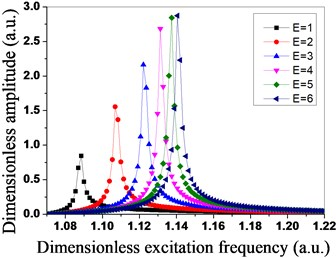Fig. 7Total strain energy distribution of tuned bladed disk system under different engine orders of excitation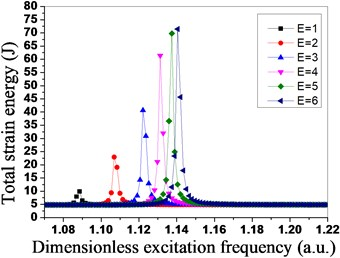Fig. 8Amplitude distribution of mistuned bladed disk system under different engine orders of excitation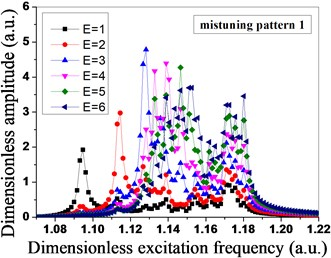a)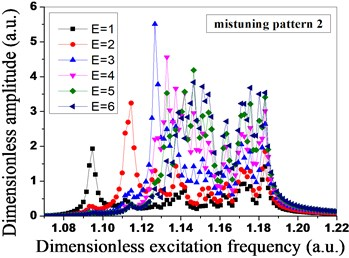b)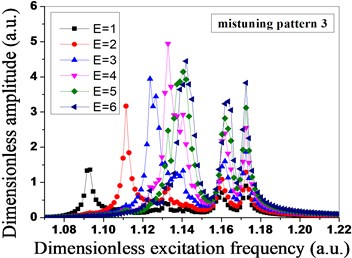c)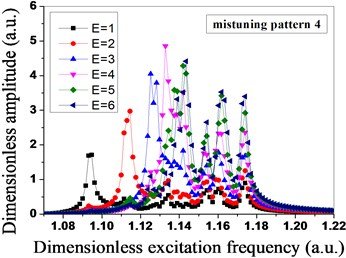d)

As shown in Fig. 8, due to the mistuned mode 1 and mistuned mode 2 are in the same group, mistuned mode 3 and mistuned mode 4 are in the same group, the vibration amplitude of the mistuned mode 1 and the vibration amplitude of the mistuned mode 2 are the maximum when the engine order of excitation is 3, however, the vibration amplitude of the mistuned mode 3 and the vibration amplitude of the mistuned mode 4 are the maximum when the engine order of excitation is 4, the different maximum vibration amplitude of the bladed disk system is caused by the different blade mistuned modes under the different engine orders of excitation, and it shows that the level of sensitivity of the different engine orders of excitation to blade mistuning is different. The vibration amplitude is the maximum when the mode of vibration of the mistuned mode 1 and the mistuned mode 2 expends to 3 nodal diameters, and the vibration amplitude is the maximum when the mode of vibration of the mistuned mode 3 and the mistuned mode 4 expends to 4 nodal diameters.

In Fig. 9, the mistuned mode has significant influences on the total strain energy of the mistuned bladed disk system under the different engine orders of excitation. The mistuned mode 1 and mistuned mode 3 are relatively sensitive to the engine order of excitation 4, the total strain energy of the bladed disk system reaches the maximum at the moment, and the mistuned mode 2 and mistuned mode 4 are relatively sensitive to the engine order of excitation 3. In the mistuned mode 2, when the engine order of excitation is 3, the maximum total strain energy of the bladed disk system is three times that of the bladed disk system under other three mistuned modes, so for the mistuned mode 2, the bladed disk system is the most sensitive to the engine order of excitation 3. Meanwhile, the dimensionless excitation frequency of the mistuned mode 1 and mistuned mode 2 with the maximum strain energy is about 1.138, the dimensionless excitation frequency of the mistuned mode 3 and mistuned mode 4 with the maximum strain energy is about 1.13, and the mistuned mode influences both the total strain energy of the bladed disk system, and the excitation frequency for the maximum total strain energy.

Fig. 9Total strain energy distribution of mistuned bladed disk system under different engine orders of excitation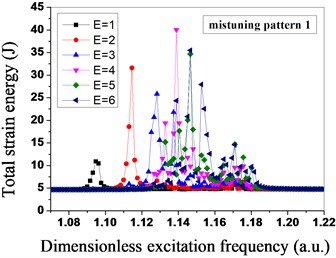a)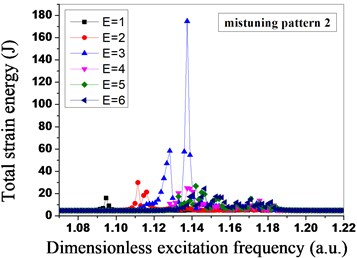b)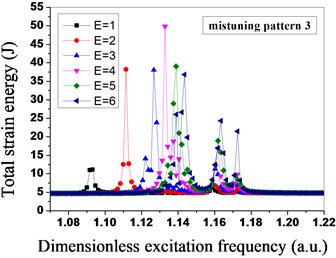c)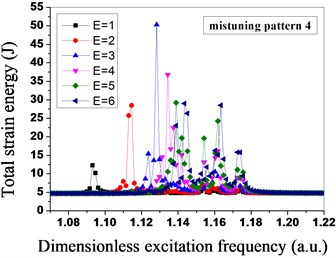d)

Fig. 10Relative localization factors of mistuned bladed disk system under different engine orders of excitation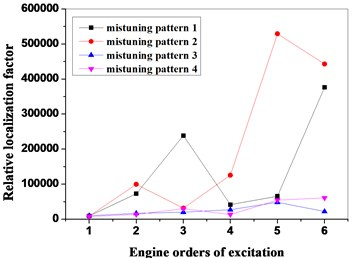As shown in Fig. 8 and Fig. 9, the mistuned mode has great influences on the vibration amplitude and the total strain energy of the bladed disk system under the different engine orders of excitation, in order to further research the influences of the mistuned modes and the different engine orders of excitation on the degree of localization of the mistuned bladed disk system, the relative localization factors of the bladed disk system in four mistuned modes under different engine orders of excitation are calculated by adopting the Eq. (19), as shown in Fig. 10. Seen from Fig. 10, under the function of the different engine orders of excitation, the relative localization factors of the mistuned mode 3 and mistuned mosde 4 of the second group are relatively smaller, and not obvious with the changes of the engine orders of excitation; however, the relative localization factors of the mistuned mode 1 and mistuned mode 2 of the first group are relative greater, and obviously change with the changes of the engine orders of excitation, and the degree of localization is relatively high.

Table 3Frequency of blades for difference from mean frequency (a.u.)

 Bladenumber Mean frequency 0.9774 0.9851 0.9927 1.0003 1.0080 1.0156 1.0232 1.0309 1 0.9466 0.9542 0.9618 0.9695 0.9771 0.9847 0.9924 1.0000 2 1.0000 1.0076 1.0153 1.0229 1.0305 1.0382 1.0458 1.0534 3 0.9450 0.9527 0.9603 0.9679 0.9756 0.9832 0.9908 0.9985 4 0.9740 0.9817 0.9893 0.9969 1.0046 1.0122 1.0198 1.0275 5 0.9618 0.9695 0.9771 0.9847 0.9924 1.0000 1.0076 1.0153 6 0.9756 0.9832 0.9908 0.9985 1.0061 1.0137 1.0214 1.0290 7 0.9634 0.9710 0.9786 0.9863 0.9939 1.0015 1.0092 1.0168 8 1.0153 1.0229 1.0305 1.0382 1.0458 1.0534 1.0611 1.0687 9 0.9618 0.9695 0.9771 0.9847 0.9924 1.0000 1.0076 1.0153 10 0.9740 0.9817 0.9893 0.9969 1.0046 1.0122 1.0198 1.0275 11 0.9618 0.9695 0.9771 0.9847 0.9924 1.0000 1.0076 1.0153 12 0.9771 0.9847 0.9924 1.0000 1.0076 1.0153 1.0229 1.0305 13 0.9634 0.9710 0.9786 0.9863 0.9939 1.0015 1.0092 1.0168 14 1.0015 1.0092 1.0168 1.0244 1.0321 1.0397 1.0473 1.0550 15 0.9481 0.9557 0.9634 0.9710 0.9786 0.9863 0.9939 1.0015 16 1.0015 1.0092 1.0168 1.0244 1.0321 1.0397 1.0473 1.0550 17 0.9740 0.9817 0.9893 0.9969 1.0046 1.0122 1.0198 1.0275 18 0.9863 0.9939 1.0015 1.0092 1.0168 1.0244 1.0321 1.0397 19 0.9725 0.9802 0.9878 0.9954 1.0031 1.0107 1.0183 1.0260 20 0.9847 0.9924 1.0000 1.0076 1.0153 1.0229 1.0305 1.0382 21 0.9725 0.9802 0.9878 0.9954 1.0031 1.0107 1.0183 1.0260 22 0.9863 0.9939 1.0015 1.0092 1.0168 1.0244 1.0321 1.0397 23 0.9725 0.9802 0.9878 0.9954 1.0031 1.0107 1.0183 1.0260 24 0.9847 0.9924 1.0000 1.0076 1.0153 1.0229 1.0305 1.0382 25 0.9710 0.9786 0.9863 0.9939 1.0015 1.0092 1.0168 1.0244 26 1.0076 1.0153 1.0229 1.0305 1.0382 1.0458 1.0534 1.0611 27 0.9557 0.9634 0.9710 0.9786 0.9863 0.9939 1.0015 1.0092 28 1.0061 1.0137 1.0214 1.0290 1.0366 1.0443 1.0519 1.0595 29 0.9786 0.9863 0.9939 1.0015 1.0092 1.0168 1.0244 1.0321 30 0.9908 0.9985 1.0061 1.0137 1.0214 1.0290 1.0366 1.0443 31 0.9740 0.9817 0.9893 0.9969 1.0046 1.0122 1.0198 1.0275 32 1.0031 1.0107 1.0183 1.0260 1.0336 1.0412 1.0489 1.0565 33 0.9496 0.9573 0.9649 0.9725 0.9802 0.9878 0.9954 1.0031 34 0.9863 0.9939 1.0015 1.0092 1.0168 1.0244 1.0321 1.0397 35 0.9725 0.9802 0.9878 0.9954 1.0031 1.0107 1.0183 1.0260 36 0.9847 0.9924 1.0000 1.0076 1.0153 1.0229 1.0305 1.0382 37 0.9725 0.9802 0.9878 0.9954 1.0031 1.0107 1.0183 1.0260 38 0.9847 0.9924 1.0000 1.0076 1.0153 1.0229 1.0305 1.0382

### 4.2. Localization influences of mean frequency of different blades on mistuned bladed disk system

The vibration response of the mistuned bladed disk system is analyzed through the mean frequency of eight groups of blades in Table 3, and the analysis result is shown in Fig. 11 to Fig. 14. The relative localization characteristics of the bladed disk system under different mean frequencies are shown in Fig. 11, because the peak value is between 1.000321 and 1.007955 of the mean frequency of the blades, in order to find out the mean frequency of the blades corresponding to the real peak value, four groups of dimensionless mean frequencies are added into the interval of 0.00153 of the blade frequency between two mean frequencies, when the mean frequency is 1.00185 by calculation, the relative localization factor of the bladed disk system is the maximum, and the degree of localization is the highest.

Fig. 11Relative localization characteristics of bladed disk system under different mean frequencies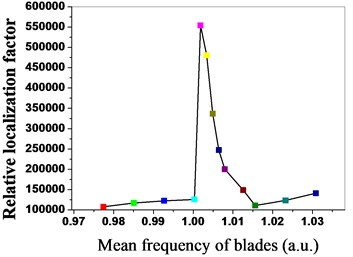Fig. 12Amplitude-frequency characteristics of bladed disk system under mean frequency is 1.00185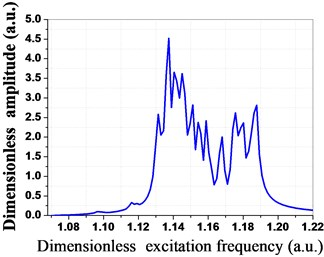Fig. 13Amplitude of mean frequency is 1.00185 for every mistuned bladed disk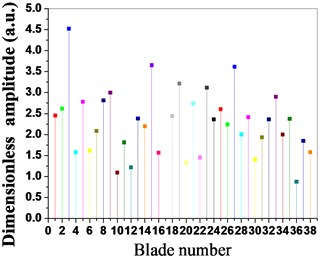Fig. 14Strain energy of mean frequency is 1.00185 for every mistuned bladed disk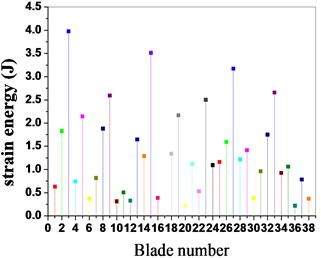### 4.3. Influences of different blades and disk stiffness ratio on mistuned bladed disk system

Fig. 15Strain energy of bladed disk system under different stiffness ratio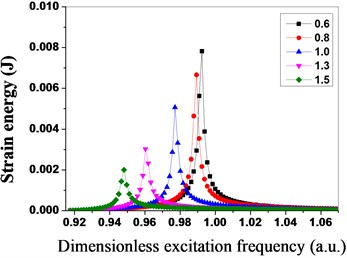Fig. 16Maximum strain energy distribution of blades under different stiffness ratio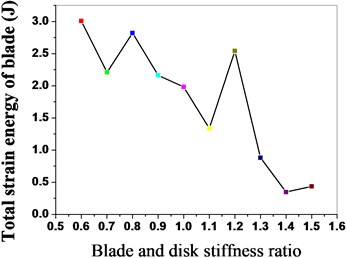Fig. 17Maximum vibration amplitude of bladed disk under different stiffness ratio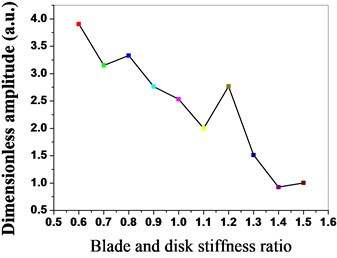Fig. 18Maximum strain energy of bladed disk under different stiffness ratio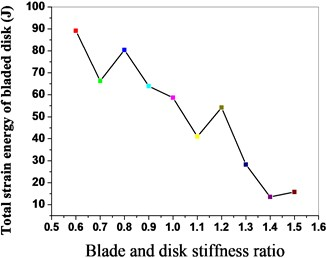As shown in Fig. 15, the maximum strain energy of the bladed disk system declines with the increase of the stiffness ratio, the strain energy of the bladed disk system is the minimum when the stiffness ratio is 1.5; meanwhile, the excitation frequency with the maximum strain energy decreases thereupon. The maximum strain energy distribution of the blades is shown in Fig. 16 when the stiffness ratio is different, and the strain energy of the blades declines with the increase of the stiffness ratio. The maximum vibration amplitude and the maximum strain energy distribution conditions of the bladed disk system are shown in Fig. 17 and Fig. 18 when the stiffness ratio is different, and the maximum vibration amplitude and maximum strain energy of the bladed disk system gradually decline with the increase of the stiffness ratio.

## 5. Conclusions

Aiming at the aero-engine compressor bladed disk system, the pre-stressed component mode synthesis method is adopted for establishing the finite element reduced-order models (ROMs), the identification of mistuned parameters through the method of combination of blade static frequency, dichotomy and finite element analysis, the localization characteristics of the vibration response of the mistuned bladed disk system are analyzed by combining the relative localization factors, the influences of different engine orders of excitation, mean frequency of the blades, and stiffness ratio between the blades and disk on localization of the vibration response of the mistuned bladed disk system, the following conclusions are obtained:

1) The pre-stressed component mode synthesis method is adopted for sharply reducing the freedom degree of the mass matrix and stiffness matrix;

2) The identification of mistuned parameters through the method of combination of blade static frequency, dichotomy and finite element analysis to accurately simulate the actual mistuned bladed disk system for engineering.

3) The relative localization factors are better evaluated than the degree of localization of vibration response of the mistuned bladed disk system between different engine orders of excitation.

4) The influences of different engine orders of excitation, the mean frequency of the blades, and the stiffness ratio between the blades and disk on the localization of vibration response of the mistuned bladed disk system are analyzed to obtain the vibration localization characteristics of the mistuned bladed disk system.

10 October 2016
Accepted
21 February 2017
Published
15 August 2017
SUBJECTS
Fault diagnosis based on vibration signal analysis
Keywords